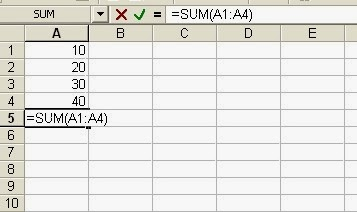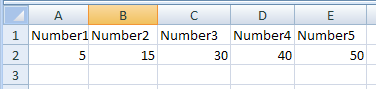# Practical Use of SUM Function in Excel Worksheet

By | February 10, 2014

### What is SUM Function?

The SUM Function is used to add all numbers in a given range of cells and returns the total as a result. The syntax of the SUM Function is as follows:

#### SUM(FirstCell : LastCell)

where FirstCell is the cell reference of the first cell in cell range and LastCell is the cell reference of the last cell in the given cell range to be added.
For Example: If cells A1,A2,A3,A4 contains 10,20,30,40 respectively then the function =SUM(A1:A4) will give 100.Simple Use of SUM function in MS Example with examples

### Syntax 2 of SUM Function in Excel Worksheet:

SUM(number1, number2, number3, …, number N)
For Example:
= SUM(10,20,30)
will give the result 60.   and  = SUM(2,5) will give 7.

### Syntax 3 of SUM Function in Excel Worksheets:

SUM(cell1, cell2, cell3, . . ., cell N)
Where cell1, cell2, cell3,…cell N are the cell references of the cells containing numbers to be added.
For Example: =SUM(A1, A3,A5) will give 10 if cell A1, A3, A5 have 2,3,5 respectively.There are some more possibilities to use the SUM function as following:If cells A2:E2 contain 5, 15, 30, 40, and 50 as show in above Excel Worksheet image:
SUM(A2:C2) equals 50        because 5+15+30
and SUM(B2:E2, 15) equals 150    because 15+30+40+50   and +15

If A1:A4 contains 10,20,30,40 respectively and cell A5 contains a non numeric value like “Excel” then the function = SUM(A1:A5) will return 100 because it ignores non-numeric value in Cell A5 that is Excel.